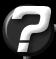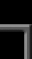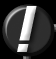Point-Plane Collision Question submitted by (07 June 2000)Return to The ArchivesI am currently writting a 3D game engine and have decided that collision detection with the ground should not be all that difficult. I have basically setup a grid of tiles made from 2 polygons. the height is measured in the Y axis. At any givin time I know my current X and Z value in the grid..From this I can get the three points that make up the polygon im in. My question is after knowing my X and z value and having the three points for the plane im in. How can I solve the plane equation to find where my Y value(height) should be to place me on the plane. The tiles are not complex curved surfaces just plane traingles.You can use the ray-plane intersections for this. This is better than simply solving for Y, since you'll get more flexibility from the ray/plane intersections as your engine grows.

The following routine receives (as input) the Ray's origin (the point you're using to test for collisions) and direction (the direction your object is headed - if you just want straight-down collision, set this to [0, -1.0, 0]). It also receives the plane's origin (any point on the plane - if it's a polygon, any vertex will do) and the plane's normal (the polygon's surface normal). The result of this routine is not the actual collision point, but rather a value often referred to as "time"; the distance from the ray's origin to the point of collision.

 ``` double intersect(Point rOrigin, Vector rNormal, Point pOrigin, Vector pNormal) { // Calc D for the plane (this is usually pre-calculated // and stored with the plane) double D = dotProduct(pOrigin, pNormal); // Determine the distance from rOrigin to the point of // intersection on the plane double numer = dotProduct(pNormal, rOrigin) + D; double denom = dotProduct(pNormal, rNormal); return -(numer / denom); } ```

If you want the actual point of collision, call this routine (which references the routine above):

 ``` Point collide(Point rOrigin, Vector rNormal, Point pOrigin, Vector pNormal) { // Get the distance to the collision point double time = intersect(rOrigin, rNormal, pOrigin, pNormal); // Calculate the collision point Point collisionPoint; collisionPoint.x = rOrigin.x + rNormal.x * time; collisionPoint.y = rOrigin.y + rNormal.y * time; collisionPoint.z = rOrigin.z + rNormal.z * time; // Here it is... return collisionPoint; } ```

And finally, in case you don't happen to have a dotProduct() routine handy...

 ``` double dotProduct(Vector v1, Vector v2) { return v1.x * v2.x + v1.y * v2.y + v1.z * v2.z; } ```

This code comes from my personal libraries which have been proven. However they have been heavily modified for sake of this example. If a mistake was made in these modifications, my apologies.

One thing to note about this code... This simply tests for intersections with the polygon's plane (which is infinite) not the polygon itself. With this, you'll never be able to fall off the edge of your world. As you progress with your engine, you'll definitely need to determine if the collision point is within the polygon, rather than simply on the polygon's plane. But this is a topic for another day.Response provided by Paul Nettle